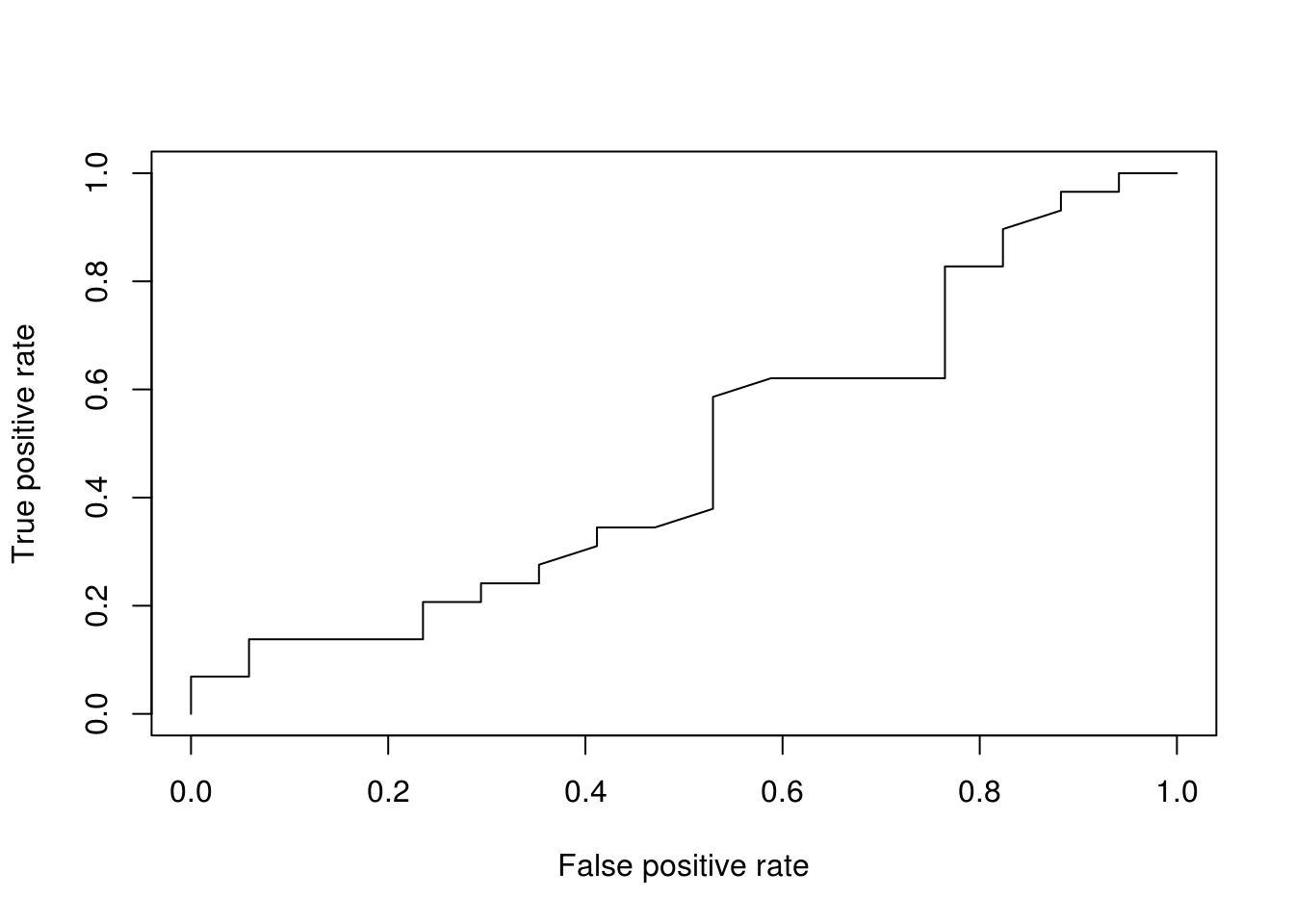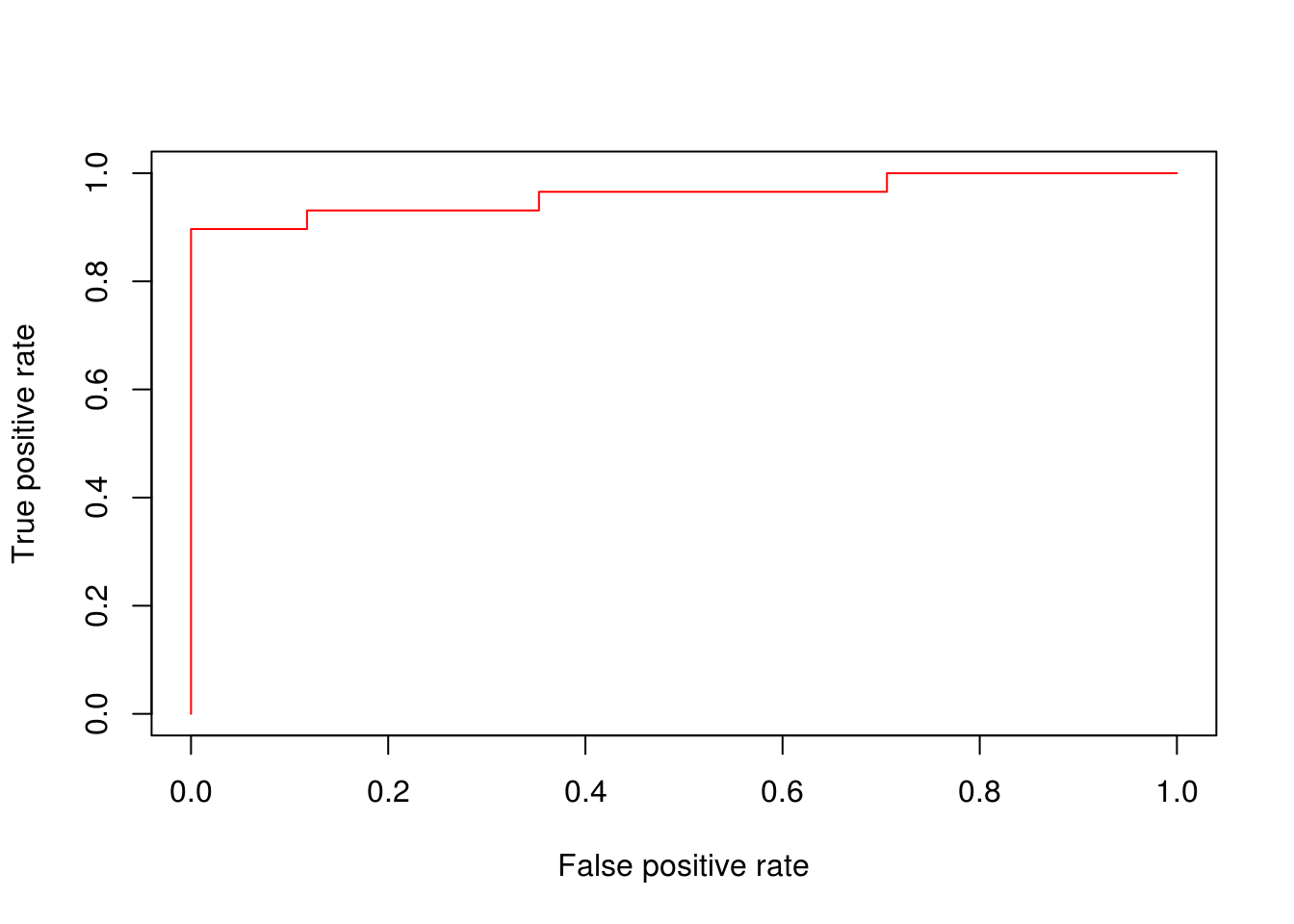# Validating a Logistic Model in R

In this post, we are going to continue our analysis of the logistic regression model from the post on logistic regression in R. Weneed to rerun all of the code from the last post to be ready to continue. As such the code form the last post is all below

``````library(MASS);library(bestglm);library(reshape2);library(corrplot);
library(ggplot2);library(ROCR)``````
``````data(survey)
survey\$Clap<-NULL
survey\$W.Hnd<-NULL
survey\$Fold<-NULL
survey\$Exer<-NULL
survey\$Smoke<-NULL
survey\$M.I<-NULL
survey<-na.omit(survey)
pm<-melt(survey, id.var="Sex")
ggplot(pm,aes(Sex,value))+geom_boxplot()+facet_wrap(~variable,ncol = 3)``````

```pc<-cor(survey[,2:5]) corrplot.mixed(pc)```

`set.seed(123) ind<-sample(2,nrow(survey),replace=T,prob = c(0.7,0.3)) train<-survey[ind==1,] test<-survey[ind==2,] fit<-glm(Sex~.,binomial,train) exp(coef(fit))`

``````train\$probs<-predict(fit, type = 'response')
train\$predict<-rep('Female',123)
train\$predict[train\$probs>0.5]<-"Male"
table(train\$predict,train\$Sex)``````
``mean(train\$predict==train\$Sex)``
``````test\$prob<-predict(fit,newdata = test, type = 'response')
test\$predict<-rep('Female',46)
test\$predict[test\$prob>0.5]<-"Male"
table(test\$predict,test\$Sex)``````
``mean(test\$predict==test\$Sex)``

Model Validation

We will now do a K-fold cross validation in order to further see how our model is doing. We cannot use the factor variable “Sex” with the K-fold code so we need to create a dummy variable. First, we create a variable called “y” that has 123 spaces, which is the same size as the “train” dataset. Second, we fill “y” with 1 in every example that is coded “male” in the “Sex” variable.

In addition, we also need to create a new dataset and remove some variables from our prior analysis otherwise we will confuse the functions that we are going to use. We will remove “predict”, “Sex”, and “probs”

``````train\$y<-rep(0,123)
train\$y[train\$Sex=="Male"]=1
my.cv<-train[,-8]
my.cv\$Sex<-NULL
my.cv\$probs<-NULL``````

We now can do our K-fold analysis. The code is complicated so you can trust it and double check on your own.

``bestglm(Xy=my.cv,IC="CV",CVArgs = list(Method="HTF",K=10,REP=1),family = binomial)``
``## Morgan-Tatar search since family is non-gaussian.``
``````## CV(K = 10, REP = 1)
## BICq equivalent for q in (6.66133814775094e-16, 0.0328567092272112)
## Best Model:
##                Estimate Std. Error   z value     Pr(>|z|)
## (Intercept) -45.2329733 7.80146036 -5.798014 6.710501e-09
## Height        0.2615027 0.04534919  5.766425 8.097067e-09``````

The results confirm what we alreaedy knew that only the “Height” variable is valuable in predicting Sex. We will now create our new model using only the recommendation of the kfold validation analysis. Then we check the new model against the train dataset and with the test dataset. The code below is a repeat of prior code but based on the cross-validation

``````reduce.fit<-glm(Sex~Height, family=binomial,train)
train\$cv.probs<-predict(reduce.fit,type='response')
train\$cv.predict<-rep('Female',123)
train\$cv.predict[train\$cv.probs>0.5]='Male'
table(train\$cv.predict,train\$Sex)``````
``````##
##          Female Male
##   Female     61   11
##   Male        7   44``````
``mean(train\$cv.predict==train\$Sex)``
``##  0.8536585``
``````test\$cv.probs<-predict(reduce.fit,test,type = 'response')
test\$cv.predict<-rep('Female',46)
test\$cv.predict[test\$cv.probs>0.5]='Male'
table(test\$cv.predict,test\$Sex)``````
``````##
##          Female Male
##   Female     16    7
##   Male        1   22``````
``mean(test\$cv.predict==test\$Sex)``
``##  0.826087``

The results are consistent for both the train and test dataset. We are now going to create the ROC curve. This will provide a visual and the AUC number to further help us to assess our model. However, a model is only good when it is compared to another model. Therefore, we will create a really bad model in order to compare it to the original model, and the cross validated model. We will first make a bad model and store the probabilities in the “test” dataset. The bad model will use “age” to predict “Sex” which doesn’t make any sense at all. Below is the code followed by the ROC curve of the bad model.

``````bad.fit<-glm(Sex~Age,family = binomial,test)The more of a diagonal the line is the worst it is. As we can see the bad model is really bad.

What we just did with the bad model we will now repeat for the full model and the cross-validated model.  As before, we need to store the prediction in a way that the ROCR package can use them. We will create a variable called “pred.full” to begin the process of graphing the original full model from the last blog post. Then we will use the “prediction” function. Next, we will create the “perf.full” variable to store the performance of the model. Notice, the arguments ‘tpr’ and ‘fpr’ for true positive rate and false positive rate. Lastly, we plot the results

``````pred.full<-prediction(test\$prob,test\$Sex)
perf.full<-performance(pred.full,'tpr','fpr')
plot(perf.full, col=2)``````We repeat this process for the cross-validated model

``````pred.cv<-prediction(test\$cv.probs,test\$Sex)
perf.cv<-performance(pred.cv,'tpr','fpr')
plot(perf.cv,col=3)``````Now let’s put all the different models on one plot

``````plot(perf.bad,col=1)Finally, we can calculate the AUC for each model

``````auc.bad<-performance(pred.bad,'auc')
``````## []
``````auc.full<-performance(pred.full,"auc")
``````## []
``````auc.cv<-performance(pred.cv,'auc')
``````## []